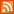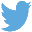Bucaro TecHelpHTTPS Encryption not required because no account numbers orpersonal information is ever requested or accepted by this siteCustom Search# Use JavaScript parseInt() and parseFloat() to Convert Strings to Numbers

When you use different data types in operations, JavaScript tries it's best to perform the proper data type conversions to make the operation work, but sometimes it can't figure out what you have in mind. Take for example the simple addition operation shown below.

4 + "4"

You might assume the result would be the number 8, but instead the result would be the text string "44". Another example is shown below.

4.4 + "4.4"

You might assume the result would be the number 8.8, but instead the result would be the text string "4.44.4".

If the results of a mathematical operation in your script are not what you expected, make sure that you convert any numbers represented as strings to the actual number data type before performing the operation.

JavaScript provides two built-in functions to convert numbers represented as strings to actual numbers, parseInt() and parseFloat().

parseInt()

parseInt() converts an integer represented as string to the number data type. An integer is a whole number with no decimal point. The statement shown below shows the previous operation using parseInt().

4 + parseInt("4")

This time the result would be the number 8 as expected.

parseFloat()

parseFloat() converts a floating-point number represented as string to the number data type. The statement shown below shows the previous operation using parseFloat().

4.4 + parseFloat("4.4")

This time the result would be the number 8.8 as expected.

Some Other Useful JavaScript Built-in Functions for Determining Data Type

typeof() Function

The typeof() function returns a string indicating the data type of a literal or variable passed to it. In the example below the message box would display string.

var result = 4.4 + "4.4";

In the example below the message box would display number.

var result = 4.4 + parseFloat("4.4");

isNaN() Function

The isNaN() function returns a boolean indicating if a literal or variable passed to it is an illegal number. It returns true if the value is NaN (Not-a-Number), and false if it is a number. In the example below the message box would display true (result is not a number).

var result = 4.4 + "4.4";
 .menubtn { margin-top:10px; margin-left:50px; width:220px; opacity:1; border-style:solid; border-color:#009000; background-color:#66ff66; transition-property: opacity; transition-duration: 1s; transition-timing-function: ease-out; } .menubtn:hover { opacity:1; background-color:#e5ff23; } Menu - More Java Script ProgrammingRSS FeedFollow @Stephen Bucaro